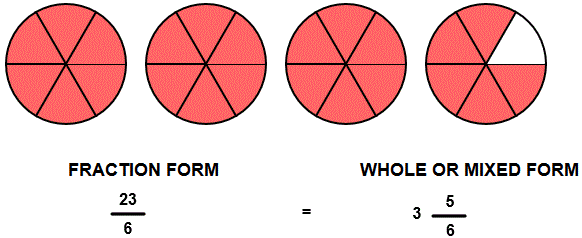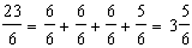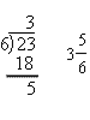# MIXED FRACTIONS WITH CIRCLES INSTRUCTIONS

Follow the directions in the dialog box after pressing the <START> button. The <EXPLAIN> button may be pressed to see how to do the example.

Identify Mixed Numbers uses circle models to demonstrate the meaning of whole number, numerator and denominator.

The illustration below was by Identify Mixed Numbers with Circles Designer.

The circles show the fraction 236. You are to write the fraction in mixed number form with a whole number, numerator, and denominator.

Each whole numbers is represented by a complete filled-in circle. Enter 3 for the whole number because there are three filled circles. The numerator is 5 because there are 5 filled-in parts in the partial circle. The denominator is 6 because there are 6 equal parts in the partial circle.Other methods can be used to arrive at the answer. One method is to see how many units of 66 there are in the fraction 236. In this case there are 3 groups of 66 in 236 giving a whole number 3. After 3 units of 66 there are 5 more parts, giving a numerator of 5. The denominator is 6 because there are 6 equal parts in a complete circle.  This method can be seen by the picture and by the illustration below:The other method is to divide the numerator by the denominator. The quotient is three with a remainder of 5, giving a whole number of 3, a numerator of 5, and a denominator of 6. This method is illustrated below:For more instruction on identifying mixed fractions go to How To Rename From Fraction Form to Mixed Form.

After you enter the fraction form answer you may press the <REPORT> button. The report will ask for your name but you may submit a code for your name. This report will give same results as on the dialog box. The report may be printed or e-mailed.